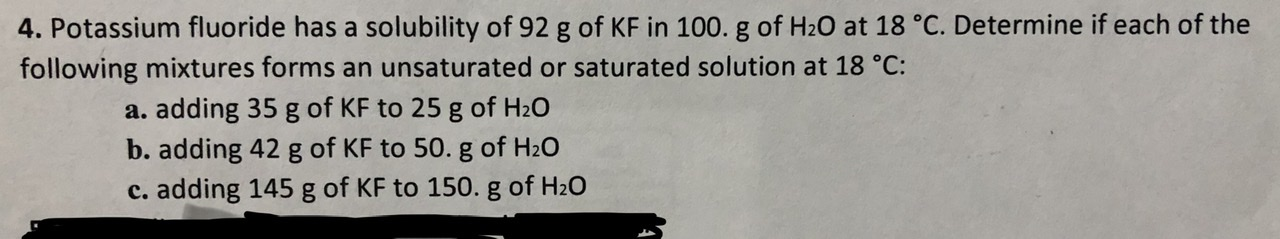# 4. Potassium fluoride has a solubility of 92 g of KF in 100. g of H20 at 18 °C. Determine if each of thefollowing mixtures forms an unsaturated or saturated solution at 18 °C:a. adding 35 g of KF to 25 g of H20b. adding 42 g of KF to 50. g of H20c. adding 145 g of KF to 150. g of H20

Question
20 viewshelp_outlineImage Transcriptionclose4. Potassium fluoride has a solubility of 92 g of KF in 100. g of H20 at 18 °C. Determine if each of the following mixtures forms an unsaturated or saturated solution at 18 °C: a. adding 35 g of KF to 25 g of H20 b. adding 42 g of KF to 50. g of H20 c. adding 145 g of KF to 150. g of H20 fullscreen
check_circle

Step 1

(a) The mixture ‘a’ contains 35 g of potassium fluoride (KF) in 25 g of water (H2O). 35 g of KF is equivalent to 25 g of H2O. The mass of KF present in 100 g of H2O (m) is calculated as shown below. The calculated mass of KF is 140 g in 100 g of H2O. The amount of KF present is more than its solubility. Hence, the mixture ‘a’ is a saturated solution.

Step 2

(b) The mixture ‘b’ contains 42 g of KF in 50 g of H2O. The mass of KF present in 100 g of H2O (m) is calculated as shown below. The calculated mass of KF is 84 ...

### Want to see the full answer?

See Solution

#### Want to see this answer and more?

Solutions are written by subject experts who are available 24/7. Questions are typically answered within 1 hour.*

See Solution
*Response times may vary by subject and question.
Tagged in

### Chemistry